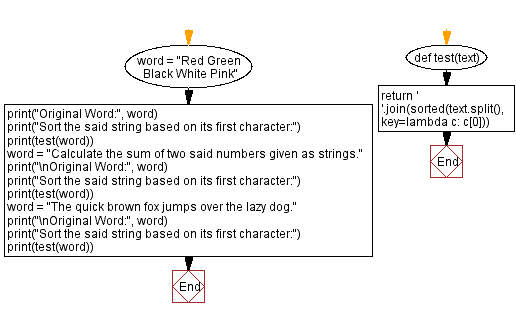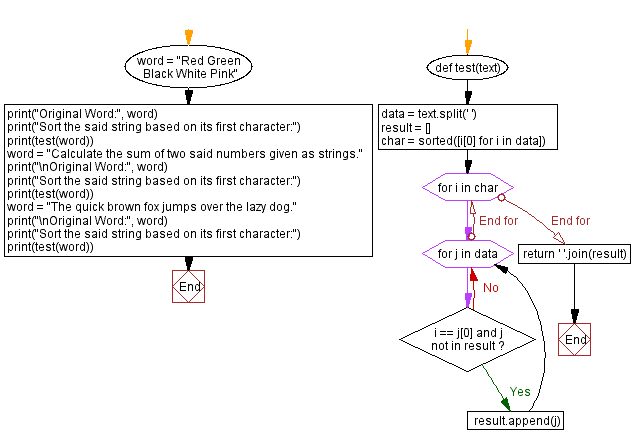﻿ Python - Sort a string based on its first character# Python Exercises: Sort a string based on its first character

## Python String: Exercise-113 with Solution

Write a Python program that returns a string sorted alphabetically by the first character of a given string of words.

Sample Data:
(“Red Green Black White Pink”) -> “Black Green Pink Red White”
(“Calculate the sum of two said numbers given as strings.”) -> (“Calculate as given numbers of sum said strings. the two”)
(“The quick brown fox jumps over the lazy dog.”) -> (“The brown dog. fox jumps lazy over quick the”)

Sample Solution-1:

Python Code:

``````def test(text):
return ' '.join(sorted(text.split(), key=lambda c: c))

word = "Red Green Black White Pink"
print("Original Word:", word)
print("Sort the said string based on its first character:")
print(test(word))
word = "Calculate the sum of two said numbers given as strings."
print("\nOriginal Word:", word)
print("Sort the said string based on its first character:")
print(test(word))
word = "The quick brown fox jumps over the lazy dog."
print("\nOriginal Word:", word)
print("Sort the said string based on its first character:")
print(test(word))
```
```

Sample Output:

```Original Word: Red Green Black White Pink
Sort the said string based on its first character:
Black Green Pink Red White

Original Word: Calculate the sum of two said numbers given as strings.
Sort the said string based on its first character:
Calculate as given numbers of sum said strings. the two

Original Word: The quick brown fox jumps over the lazy dog.
Sort the said string based on its first character:
The brown dog. fox jumps lazy over quick the
```

Flowchart:## Visualize Python code execution:

The following tool visualize what the computer is doing step-by-step as it executes the said program:

Sample Solution-2:

Python Code:

``````def test(text):
data = text.split(' ')
result = []
char = sorted([i for i in data])
for i in char:
for j in data:
if i == j and j not in result:
result.append(j)
return ' '.join(result)

word = "Red Green Black White Pink"
print("Original Word:", word)
print("Sort the said string based on its first character:")
print(test(word))
word = "Calculate the sum of two said numbers given as strings."
print("\nOriginal Word:", word)
print("Sort the said string based on its first character:")
print(test(word))
word = "The quick brown fox jumps over the lazy dog."
print("\nOriginal Word:", word)
print("Sort the said string based on its first character:")
print(test(word))
```
```

Sample Output:

```Original Word: Red Green Black White Pink
Sort the said string based on its first character:
Black Green Pink Red White

Original Word: Calculate the sum of two said numbers given as strings.
Sort the said string based on its first character:
Calculate as given numbers of sum said strings. the two

Original Word: The quick brown fox jumps over the lazy dog.
Sort the said string based on its first character:
The brown dog. fox jumps lazy over quick the
```

Flowchart:## Visualize Python code execution:

The following tool visualize what the computer is doing step-by-step as it executes the said program:

Python Code Editor:

Have another way to solve this solution? Contribute your code (and comments) through Disqus.

Previous Python Exercise: Calculate the sum of two numbers given as strings.
Next Python Exercise: Python List Exercise Home.

What is the difficulty level of this exercise?

Test your Programming skills with w3resource's quiz.

﻿

## Python: Tips of the Day

Getting sorted iterable (can sort by "compare" function):

```>>> a = [1, 2, -3]
>>> sorted(a)
[-3, 1, 2]

>>> sorted(a,key=abs)
[1, 2, -3]
```